# Basic Numerical Procedures Chapter 19 Options Futures and

• Slides: 39Basic Numerical Procedures Chapter 19 Options, Futures, and Other Derivatives, 7 th Edition, Copyright © John C. Hull 2008 1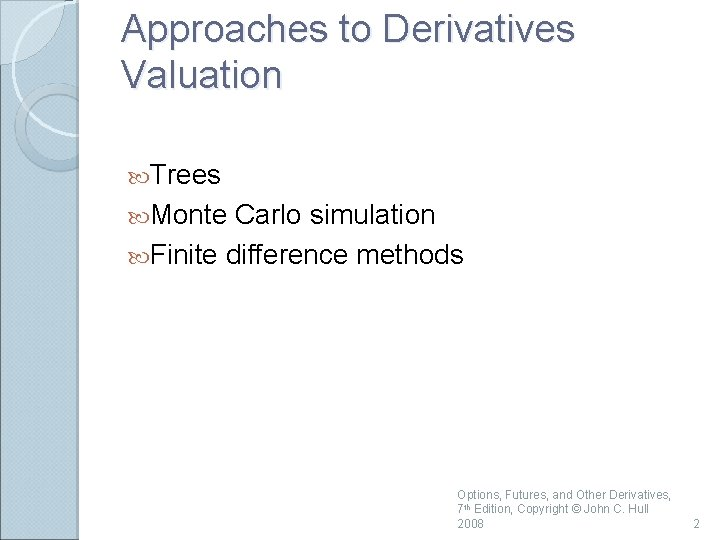Approaches to Derivatives Valuation Trees Monte Carlo simulation Finite difference methods Options, Futures, and Other Derivatives, 7 th Edition, Copyright © John C. Hull 2008 2Binomial Trees Binomial trees are frequently used to approximate the movements in the price of a stock or other asset In each small interval of time the stock price is assumed to move up by a proportional amount u or to move down by a proportional amount d Options, Futures, and Other Derivatives, 7 th Edition, Copyright © John C. Hull 2008 3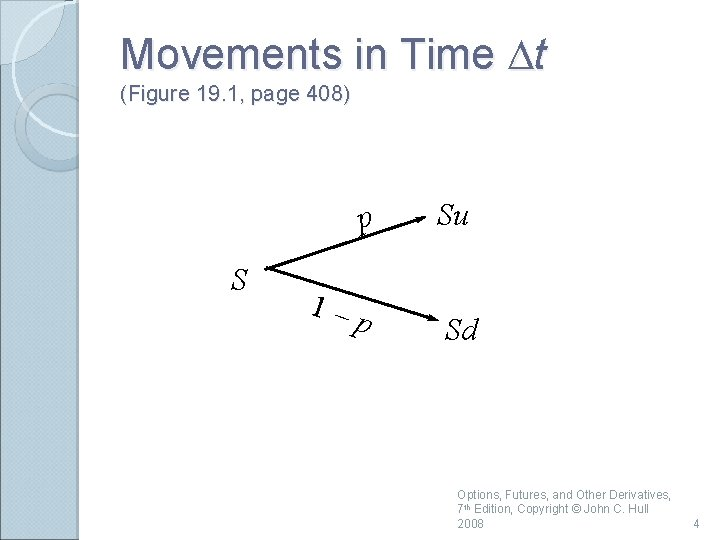Movements in Time Dt (Figure 19. 1, page 408) S p Su 1–p Sd Options, Futures, and Other Derivatives, 7 th Edition, Copyright © John C. Hull 2008 4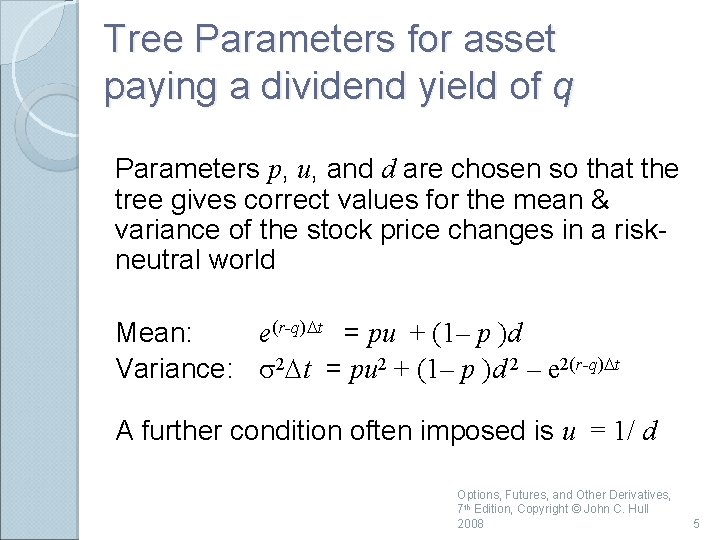Tree Parameters for asset paying a dividend yield of q Parameters p, u, and d are chosen so that the tree gives correct values for the mean & variance of the stock price changes in a riskneutral world Mean: e(r-q)Dt = pu + (1– p )d Variance: s 2 Dt = pu 2 + (1– p )d 2 – e 2(r-q)Dt A further condition often imposed is u = 1/ d Options, Futures, and Other Derivatives, 7 th Edition, Copyright © John C. Hull 2008 5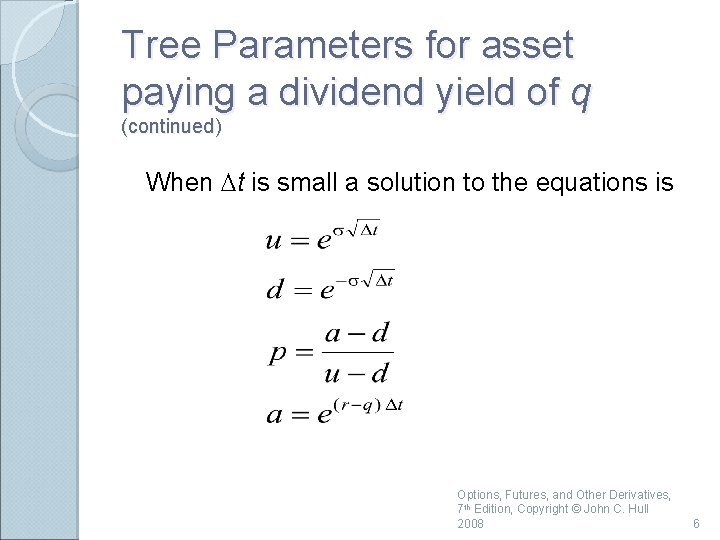Tree Parameters for asset paying a dividend yield of q (continued) When Dt is small a solution to the equations is Options, Futures, and Other Derivatives, 7 th Edition, Copyright © John C. Hull 2008 6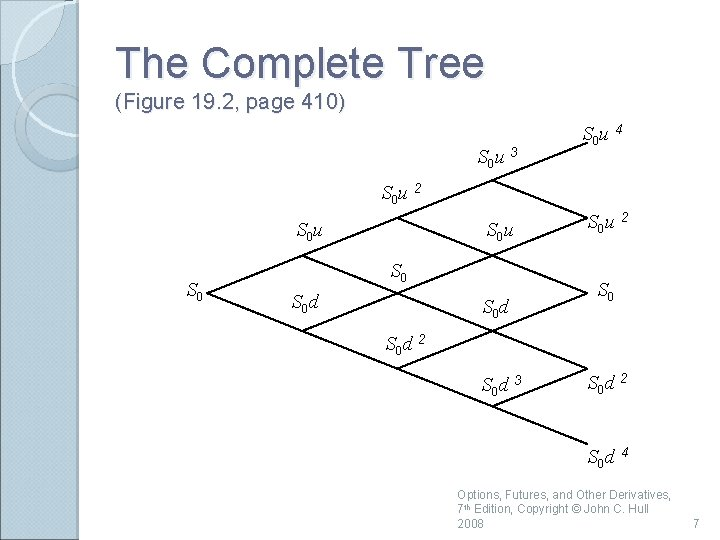The Complete Tree (Figure 19. 2, page 410) S 0 u 3 S 0 u 4 S 0 u 2 S 0 u S 0 S 0 d S 0 u 2 S 0 d 3 S 0 d 2 S 0 d 4 Options, Futures, and Other Derivatives, 7 th Edition, Copyright © John C. Hull 2008 7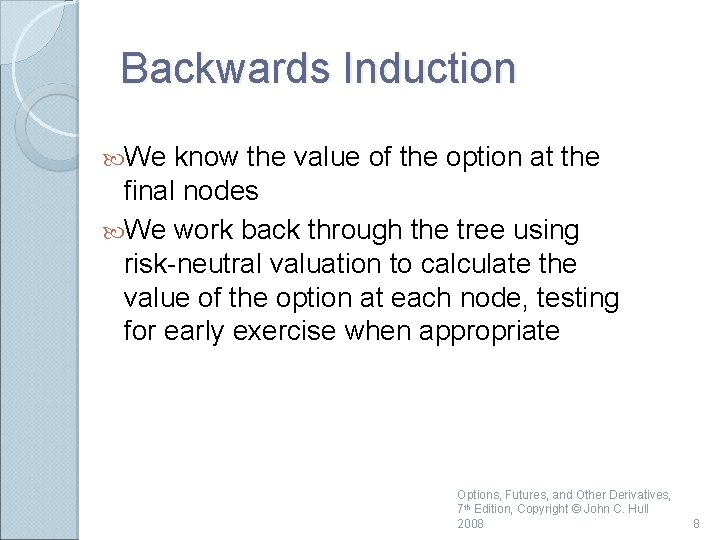Backwards Induction We know the value of the option at the final nodes We work back through the tree using risk-neutral valuation to calculate the value of the option at each node, testing for early exercise when appropriate Options, Futures, and Other Derivatives, 7 th Edition, Copyright © John C. Hull 2008 8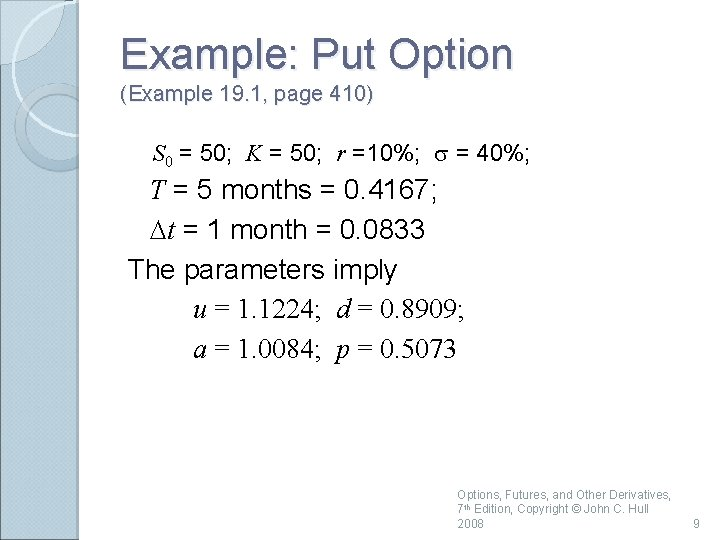Example: Put Option (Example 19. 1, page 410) S 0 = 50; K = 50; r =10%; s = 40%; T = 5 months = 0. 4167; Dt = 1 month = 0. 0833 The parameters imply u = 1. 1224; d = 0. 8909; a = 1. 0084; p = 0. 5073 Options, Futures, and Other Derivatives, 7 th Edition, Copyright © John C. Hull 2008 9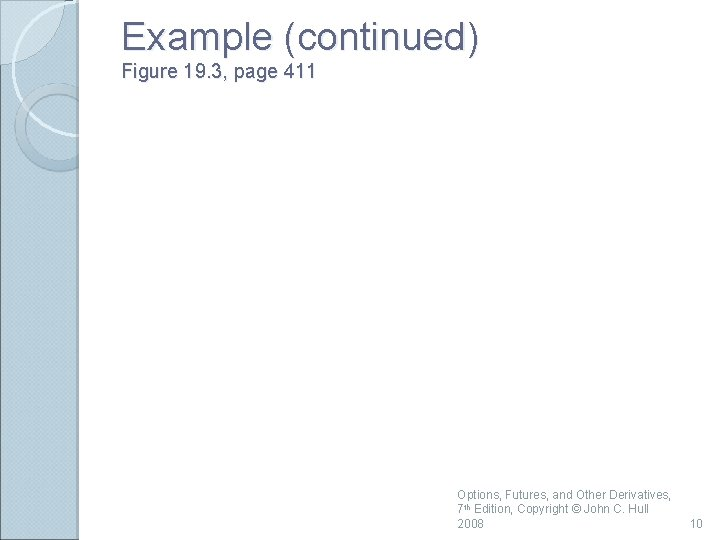Example (continued) Figure 19. 3, page 411 Options, Futures, and Other Derivatives, 7 th Edition, Copyright © John C. Hull 2008 10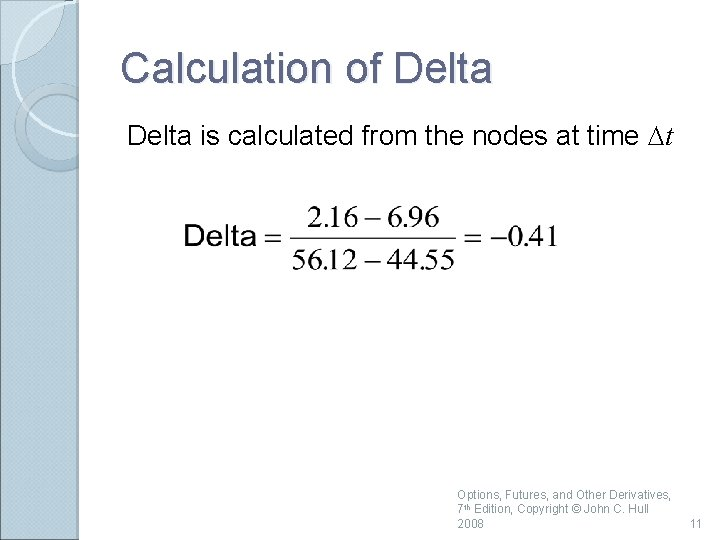Calculation of Delta is calculated from the nodes at time Dt Options, Futures, and Other Derivatives, 7 th Edition, Copyright © John C. Hull 2008 11Calculation of Gamma is calculated from the nodes at time 2 Dt Options, Futures, and Other Derivatives, 7 th Edition, Copyright © John C. Hull 2008 12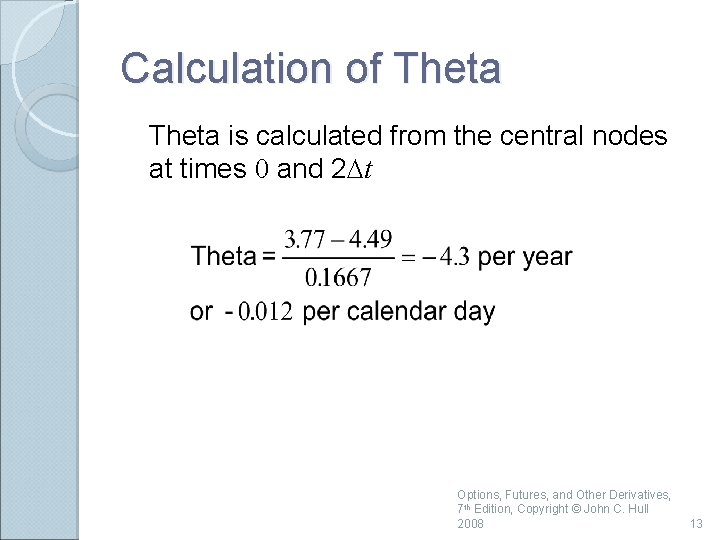Calculation of Theta is calculated from the central nodes at times 0 and 2 Dt Options, Futures, and Other Derivatives, 7 th Edition, Copyright © John C. Hull 2008 13Calculation of Vega We can proceed as follows Construct a new tree with a volatility of 41% instead of 40%. Value of option is 4. 62 Vega is Options, Futures, and Other Derivatives, 7 th Edition, Copyright © John C. Hull 2008 14Trees for Options on Indices, Currencies and Futures Contracts As with Black-Scholes: ◦ For options on stock indices, q equals the dividend yield on the index ◦ For options on a foreign currency, q equals the foreign risk-free rate ◦ For options on futures contracts q = r Options, Futures, and Other Derivatives, 7 th Edition, Copyright © John C. Hull 2008 15Binomial Tree for Stock Paying Known Dividends Procedure: ◦ Draw the tree for the stock price less the present value of the dividends ◦ Create a new tree by adding the present value of the dividends at each node This ensures that the tree recombines and makes assumptions similar to those when the Black-Scholes model is used Options, Futures, and Other Derivatives, 7 th Edition, Copyright © John C. Hull 2008 16Extensions of Tree Approach Time dependent interest rates The control variate technique Options, Futures, and Other Derivatives, 7 th Edition, Copyright © John C. Hull 2008 17Alternative Binomial Tree (Section 19. 4, page 422) Instead of setting u = 1/d we can set each of the 2 probabilities to 0. 5 and Options, Futures, and Other Derivatives, 7 th Edition, Copyright © John C. Hull 2008 18Trinomial Tree (Page 424) Su pu S pm S pd Sd Options, Futures, and Other Derivatives, 7 th Edition, Copyright © John C. Hull 2008 19Time Dependent Parameters in a Binomial Tree (page 425) Making r or q a function of time does not affect the geometry of the tree. The probabilities on the tree become functions of time. We can make s a function of time by making the lengths of the time steps inversely proportional to the variance rate. Options, Futures, and Other Derivatives, 7 th Edition, Copyright © John C. Hull 2008 20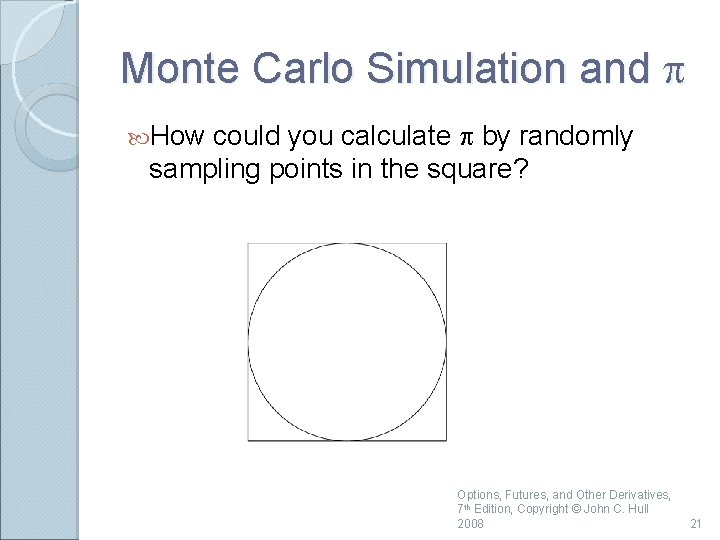Monte Carlo Simulation and p could you calculate p by randomly sampling points in the square? How Options, Futures, and Other Derivatives, 7 th Edition, Copyright © John C. Hull 2008 21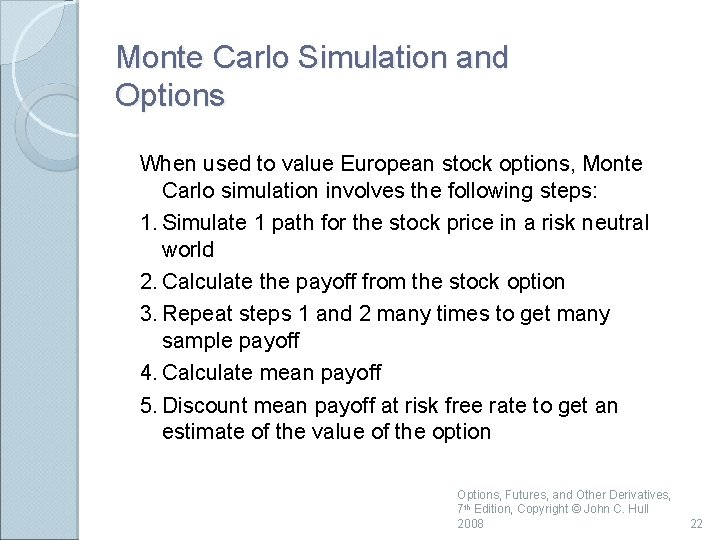Monte Carlo Simulation and Options When used to value European stock options, Monte Carlo simulation involves the following steps: 1. Simulate 1 path for the stock price in a risk neutral world 2. Calculate the payoff from the stock option 3. Repeat steps 1 and 2 many times to get many sample payoff 4. Calculate mean payoff 5. Discount mean payoff at risk free rate to get an estimate of the value of the option Options, Futures, and Other Derivatives, 7 th Edition, Copyright © John C. Hull 2008 22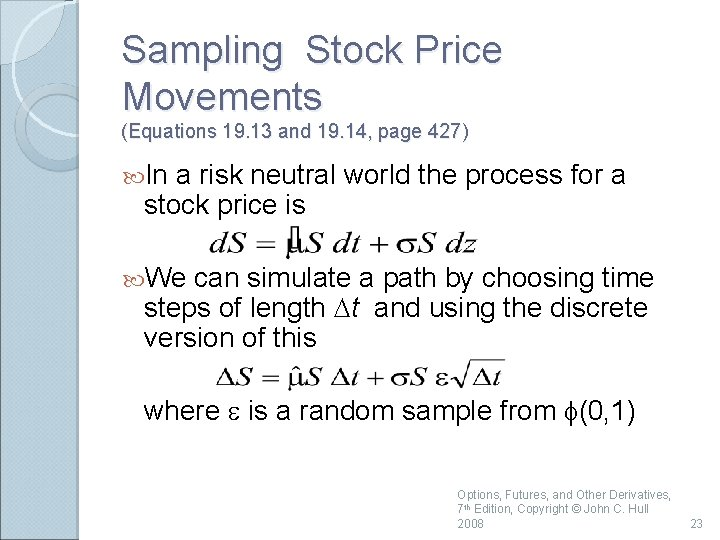Sampling Stock Price Movements (Equations 19. 13 and 19. 14, page 427) In a risk neutral world the process for a stock price is We can simulate a path by choosing time steps of length Dt and using the discrete version of this where e is a random sample from f(0, 1) Options, Futures, and Other Derivatives, 7 th Edition, Copyright © John C. Hull 2008 23A More Accurate Approach (Equation 19. 15, page 428) Options, Futures, and Other Derivatives, 7 th Edition, Copyright © John C. Hull 2008 24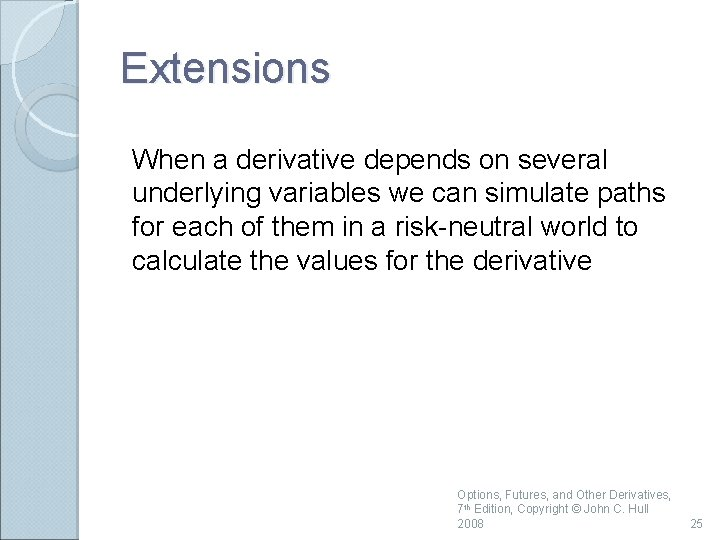Extensions When a derivative depends on several underlying variables we can simulate paths for each of them in a risk-neutral world to calculate the values for the derivative Options, Futures, and Other Derivatives, 7 th Edition, Copyright © John C. Hull 2008 25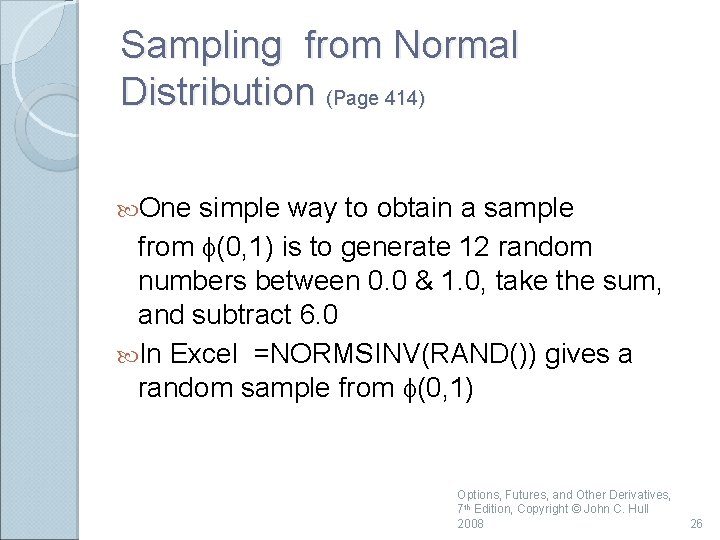Sampling from Normal Distribution (Page 414) One simple way to obtain a sample from f(0, 1) is to generate 12 random numbers between 0. 0 & 1. 0, take the sum, and subtract 6. 0 In Excel =NORMSINV(RAND()) gives a random sample from f(0, 1) Options, Futures, and Other Derivatives, 7 th Edition, Copyright © John C. Hull 2008 26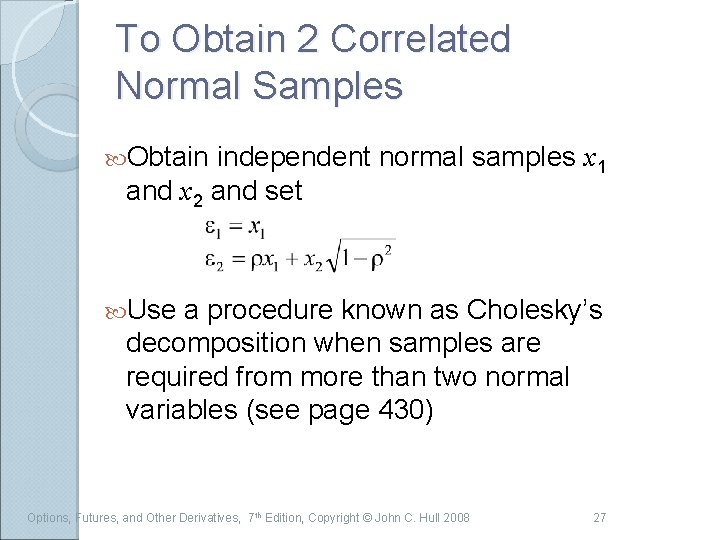To Obtain 2 Correlated Normal Samples Obtain independent normal samples x 1 and x 2 and set Use a procedure known as Cholesky’s decomposition when samples are required from more than two normal variables (see page 430) Options, Futures, and Other Derivatives, 7 th Edition, Copyright © John C. Hull 2008 27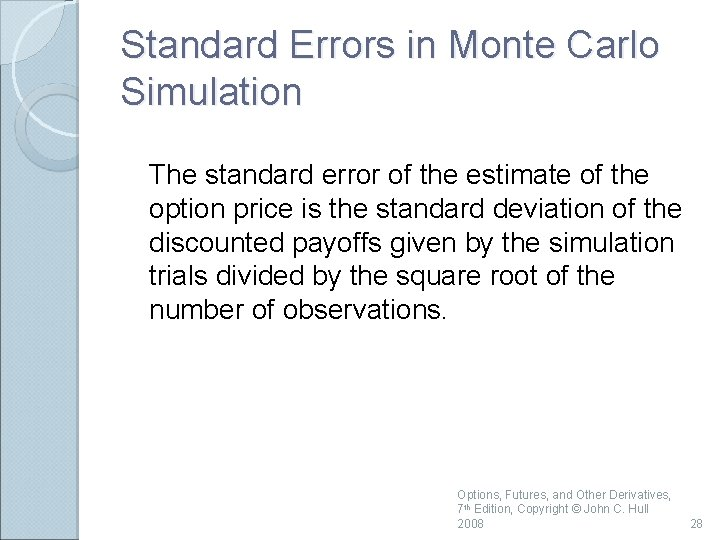Standard Errors in Monte Carlo Simulation The standard error of the estimate of the option price is the standard deviation of the discounted payoffs given by the simulation trials divided by the square root of the number of observations. Options, Futures, and Other Derivatives, 7 th Edition, Copyright © John C. Hull 2008 28Application of Monte Carlo Simulation Monte Carlo simulation can deal with path dependent options, options dependent on several underlying state variables, and options with complex payoffs It cannot easily deal with American-style options Options, Futures, and Other Derivatives, 7 th Edition, Copyright © John C. Hull 2008 29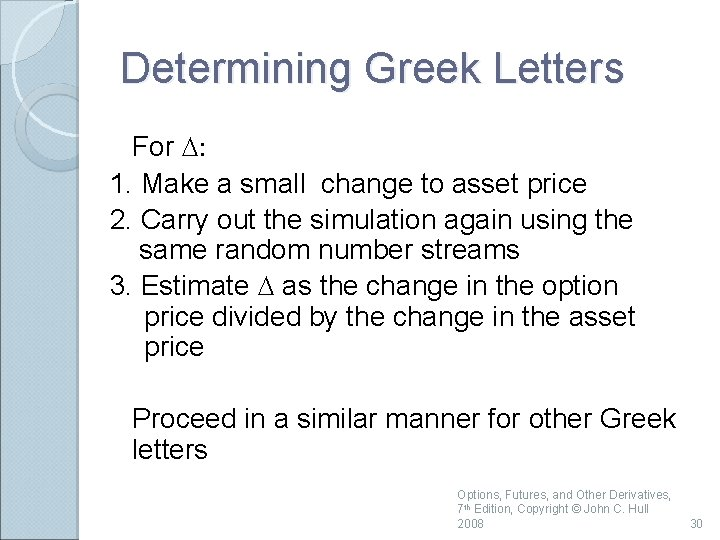Determining Greek Letters For D: 1. Make a small change to asset price 2. Carry out the simulation again using the same random number streams 3. Estimate D as the change in the option price divided by the change in the asset price Proceed in a similar manner for other Greek letters Options, Futures, and Other Derivatives, 7 th Edition, Copyright © John C. Hull 2008 30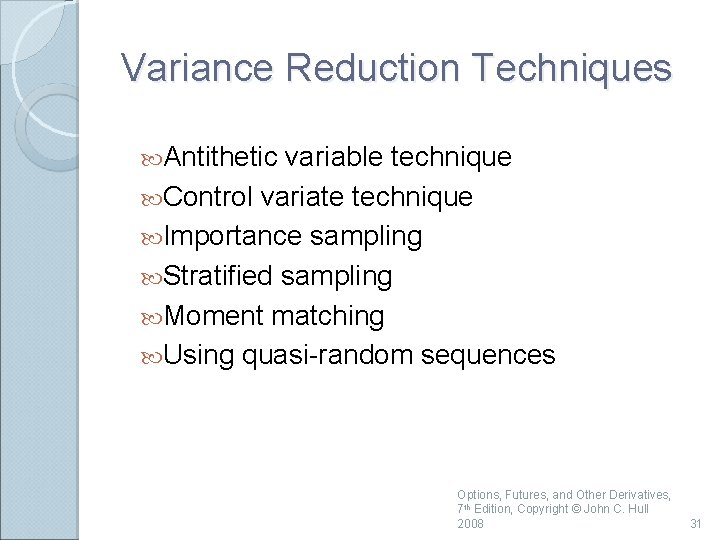Variance Reduction Techniques Antithetic variable technique Control variate technique Importance sampling Stratified sampling Moment matching Using quasi-random sequences Options, Futures, and Other Derivatives, 7 th Edition, Copyright © John C. Hull 2008 31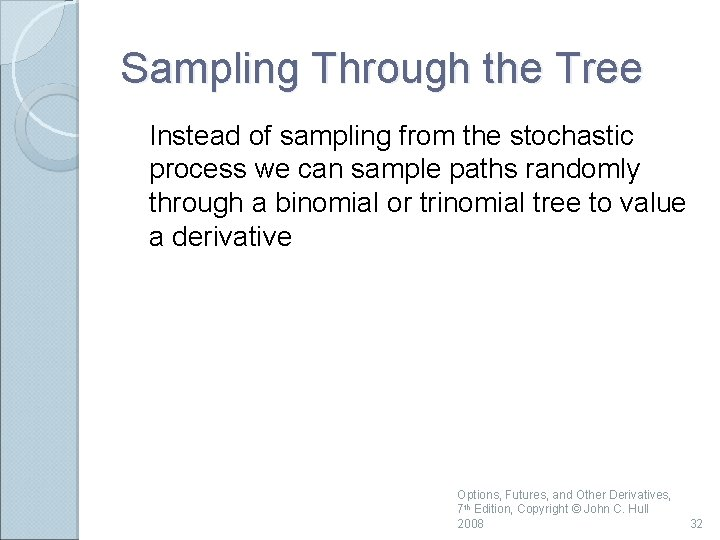Sampling Through the Tree Instead of sampling from the stochastic process we can sample paths randomly through a binomial or trinomial tree to value a derivative Options, Futures, and Other Derivatives, 7 th Edition, Copyright © John C. Hull 2008 32Finite Difference Methods Finite difference methods aim to represent the differential equation in the form of a difference equation We form a grid by considering equally spaced time values and stock price values Define ƒi, j as the value of ƒ at time i. Dt when the stock price is j. DS Options, Futures, and Other Derivatives, 7 th Edition, Copyright © John C. Hull 2008 33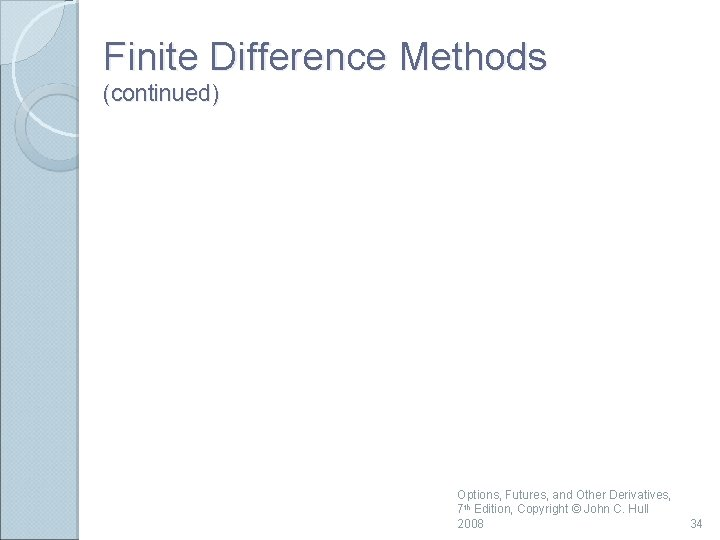Finite Difference Methods (continued) Options, Futures, and Other Derivatives, 7 th Edition, Copyright © John C. Hull 2008 34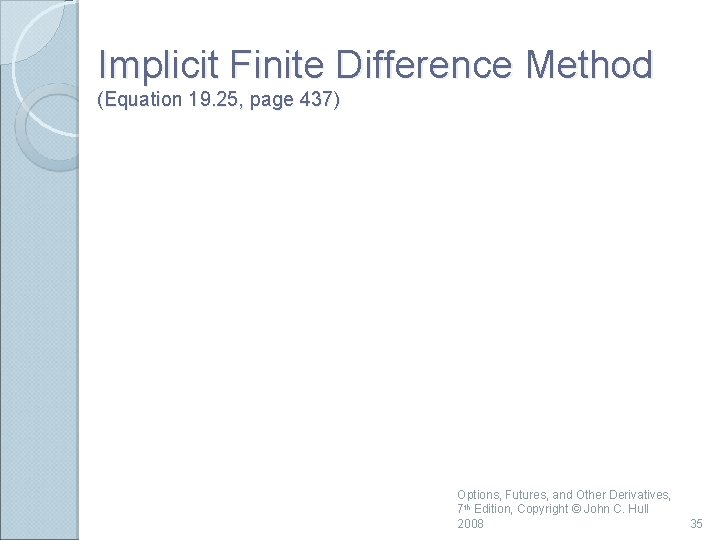Implicit Finite Difference Method (Equation 19. 25, page 437) Options, Futures, and Other Derivatives, 7 th Edition, Copyright © John C. Hull 2008 35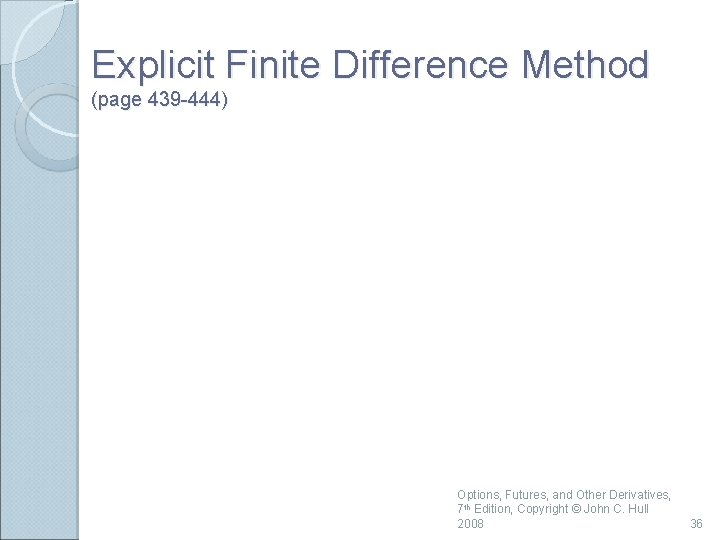Explicit Finite Difference Method (page 439 -444) Options, Futures, and Other Derivatives, 7 th Edition, Copyright © John C. Hull 2008 36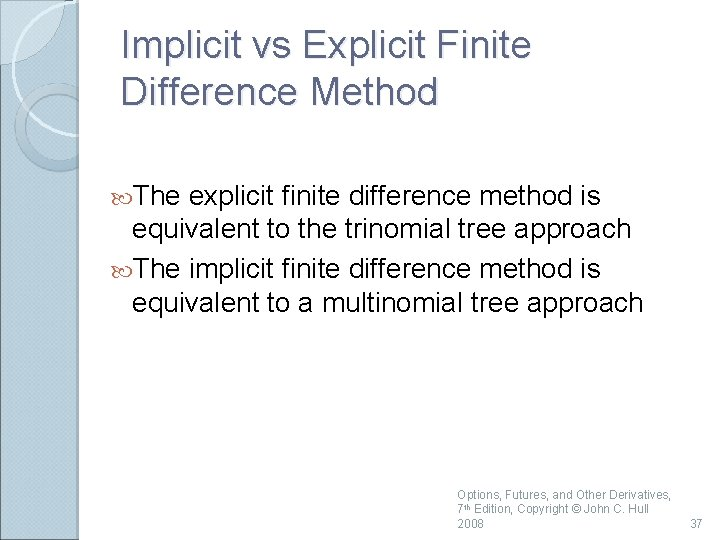Implicit vs Explicit Finite Difference Method The explicit finite difference method is equivalent to the trinomial tree approach The implicit finite difference method is equivalent to a multinomial tree approach Options, Futures, and Other Derivatives, 7 th Edition, Copyright © John C. Hull 2008 37Implicit vs Explicit Finite Difference Methods (Figure 19. 16, page 441) ƒi +1, j +1 ƒi , j ƒi +1, j ƒi , j – 1 Implicit Method ƒi +1, j – 1 Explicit Method Options, Futures, and Other Derivatives, 7 th Edition, Copyright © John C. Hull 2008 38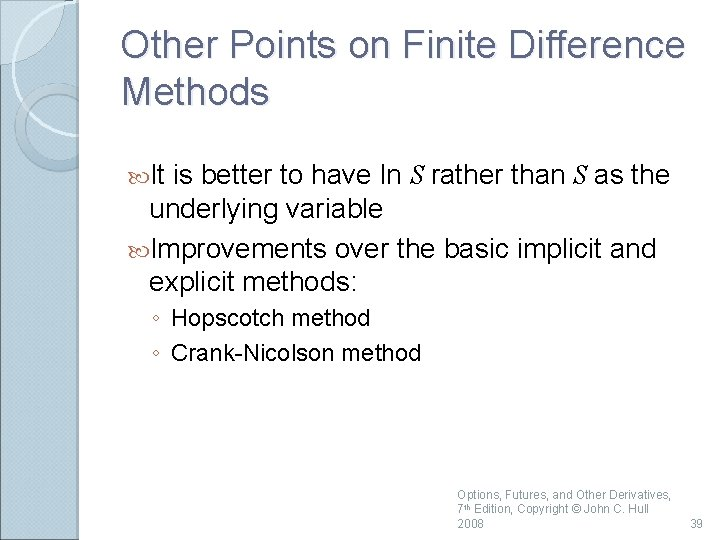Other Points on Finite Difference Methods It is better to have ln S rather than S as the underlying variable Improvements over the basic implicit and explicit methods: ◦ Hopscotch method ◦ Crank-Nicolson method Options, Futures, and Other Derivatives, 7 th Edition, Copyright © John C. Hull 2008 39